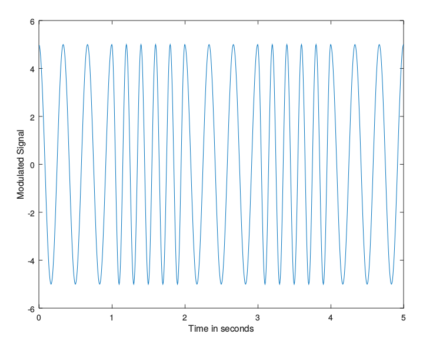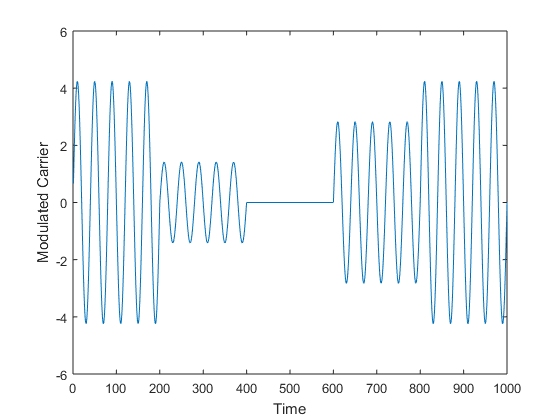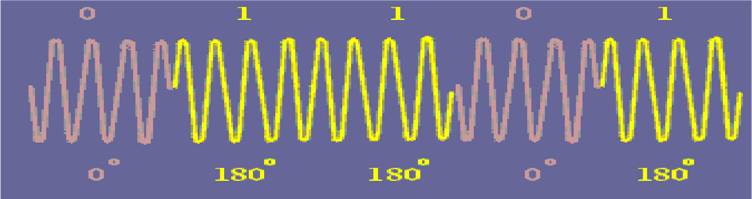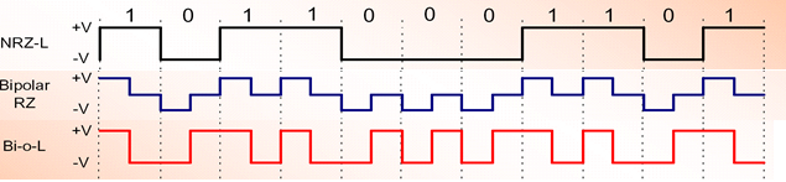# Digital Communication

## FTTH GPON Interview Questions

Q. What is FTTH? Ans. FTTH stands for Fiber to the Home Q. What is GPON? Ans. GPON stands for Gigabit Passive Optical Network. A passive optical network (PON) is a networkarchitecture that brings fiber cabling direct to the home using a point-to-multipoint scheme that enablesa single optical fiber to serve multiple premises & Customers. [...]

## Binary Frequency Shift Keying (BFSK) Modulation And Demodulation-Matlab Code With ExplanationBinary Frequency Shift Keying (BFSK) is a type of digital modulation technique in which we are sending one bit per symbol i.e., ‘0’ or a ‘1’. Hence, the bit rate and symbol rate are the same. In BFSK, the information is encoded in the variation of the frequency of the carrier. We represent ‘0’ by carrier frequency [...]

{ 1 comment }

## MASK Modulation And Demodulation-Complete Matlab Code With ExplanationBinary Amplitude Shift Keying Amplitude Shift Keying (ASK) is a type of digital modulation in which the amplitude of the carrier is varied with respect to the digital message. If we send one bit/symbol, we call it as Binary Amplitude Shift Keying (BASK), in which case the carrier amplitude is assumed to have 2 values (one corresponding to 1 [...]

{ 1 comment }

## BPSK Modulation And Demodulation- Complete Matlab Code With ExplanationBinary Phase Shift Keying (BPSK) is a type of digital modulation technique in which we are sending one bit per symbol i.e., ‘0’ or a ‘1’. Hence, the bit rate and symbol rate are the same. Depending upon the message bit, we can have a phase shift of 0o or 180o with respect to a [...]